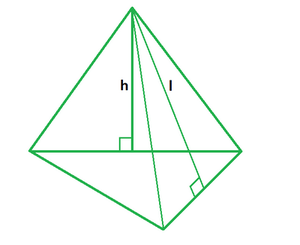Open In App

# Surface Area of a Triangular Pyramid Formula

A solid with a triangular base such that the triangles have a common vertex on all three lateral sides is called a triangular pyramid. It can be interpreted as a tetrahedron with equilateral triangles on all four faces. It is a three-dimensional object with a triangle base and four triangular faces, three of which meet at one vertex. The base of a right triangular pyramid is a right-angled triangle, while the rest of the faces are isosceles triangles.### Surface area of a Triangular pyramid

The surface area of a triangular pyramid has two formulas, that is, lateral surface area and total surface area. Let’s learn about both the formulae in more detail,

Formula for Lateral surface area of a triangular pyramid

It is calculated by adding the area of triangular faces with no base, i.e., by subtracting the base area from the total surface area. It is equal to half the product of perimeter and slant height.

L = 3/2 (b × l)

Where,

b is the side of triangular base,

l is the slant height of pyramid.

Formula for Total surface area of a triangular pyramid

It is computed by adding the area of all triangular faces of the pyramid. Its formula is equal to the sum of the base area and half the product of perimeter and slant height. It is the sum of the base area and lateral surface area of the pyramid.

A = 1/2 (b × h) + 3/2 (b × l)

Where,

b is the side of triangular base,

h is the height of triangular base,

l is the slant height of pyramid.

### Sample problems

Problem 1: Find the lateral surface area of a triangular pyramid with a base of 10 m and a slant height of 12 m.

Solution:

We have, b = 10 and l = 12

Using the formula we have,

L = 3/2 (b × l)

= 3/2 (10 × 12)

= 360/2

= 180 sq. m

Problem 2: Find the lateral surface area of a triangular pyramid with a base of 15 m and a slant height of 20 m.

Solution:

We have, b = 15 and l = 20

Using the formula we have,

L = 3/2 (b × l)

= 3/2 (15 × 20)

= 900/2

= 450 sq. m

Problem 3: Find the base length of a triangular pyramid with a slant height of 12 m and a lateral area of 180 sq. m.

Solution:

We have, l = 15 and A = 180

Using the formula we have,

L = 3/2 (b × l)

=> 180 = 3/2 (b × 12)

=> 180 = 36b/2

=> 18b = 180

=> b = 10 m

Problem 4: Find the slant length of a triangular pyramid with a base of 6 m and a lateral area of 81 sq. m.

Solution:

We have, b = 6 and A = 81

Using the formula we have,

L = 3/2 (b × l)

=> 81 = 3/2 (6 × l)

=> 81 = 18l/2

=> 9l = 81

=> l = 9 m

Problem 5: Find the total surface area of a triangular pyramid with a base of 6 m, height of 10 m, and lateral area of 100 sq. m.

Solution:

We have, b = 6, h = 10 and L = 100

Using the formula we have,

A = 1/2 (b × h) + L

= 1/2 (6 × 10) + 100

= 30 + 100

= 130 sq. m

Problem 6: Find the total surface area of a triangular pyramid with a base of 2 m, height of 4 m, and slant height of 7 m.

Solution:

We have, b = 2, h = 4 and l = 7.

Using the formula we have,

A = 1/2 (b × h) + 3/2 (b × l)

= 1/2 (2 × 4) + 3/2 (2 × 7)

= 4 + 21

= 25 sq. m

Problem 7: Find the total surface area of a triangular pyramid with a base of 16 m, height of 11 m, and slant height of 24 m.

Solution:

We have, b = 16, h = 11 and l = 24.

Using the formula we have,

A = 1/2 (b × h) + 3/2 (b × l)

= 1/2 (16 × 11) + 3/2 (16 × 24)

= 88 + 576

= 664 sq. m